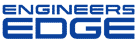# Torque Limits

[ Home ][ Forum Archive ] [ Active Engineering Forum ]

Posted By<" ">Brock Presgrove on April 30, 2002 at 09:46:53:

How do you determine the maximum allowable torque on a bolt? I reviewed the Torque Design page and don't understand how they came up with the values. For a Grade 8, 1" bolt, the affective stress area is A=0.7854 x [D-(.9743/n)]^2 = 0.606in^2, where D= nominal diameter and n= treads/inch = 8. If the allowable tensile stress is Yield x .8, (130,000 lbf/in^2)x 0.8 x(0.606in^2)= 63,024 lbf (Ref. Fastener Standards, 6th edition). Assuming the allowable shear stress is 1/2 the allowable tensile stress, 63,024/2=31,512 lbf, Max Torque = (Shear x d^3 x pie)/(16 x 12in/ft) = (31,512lbf x 0.879in x 3.1416)/(16 x 12 in/ft) = 453 ft-lbf where d is the affective diameter of the bolt (Ref. Pytel & Singer,Strength of Material, 4th edition, Chapter 3). 453 ft-lbf vs. 700 ft-lbf from Torque Design page. Why the difference?

< "> Subject: Re: Torque Limits